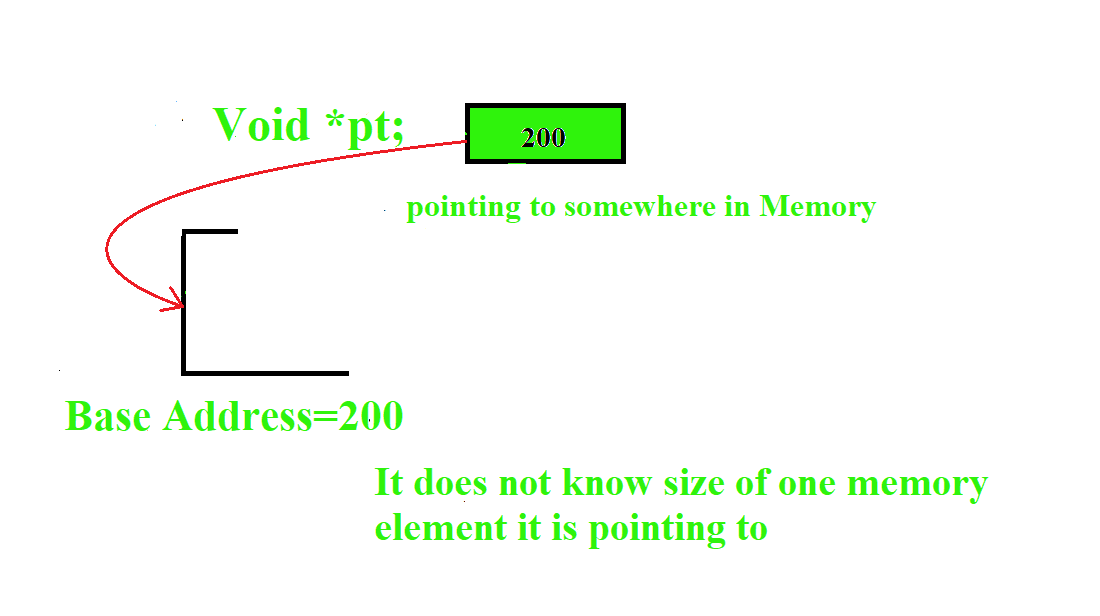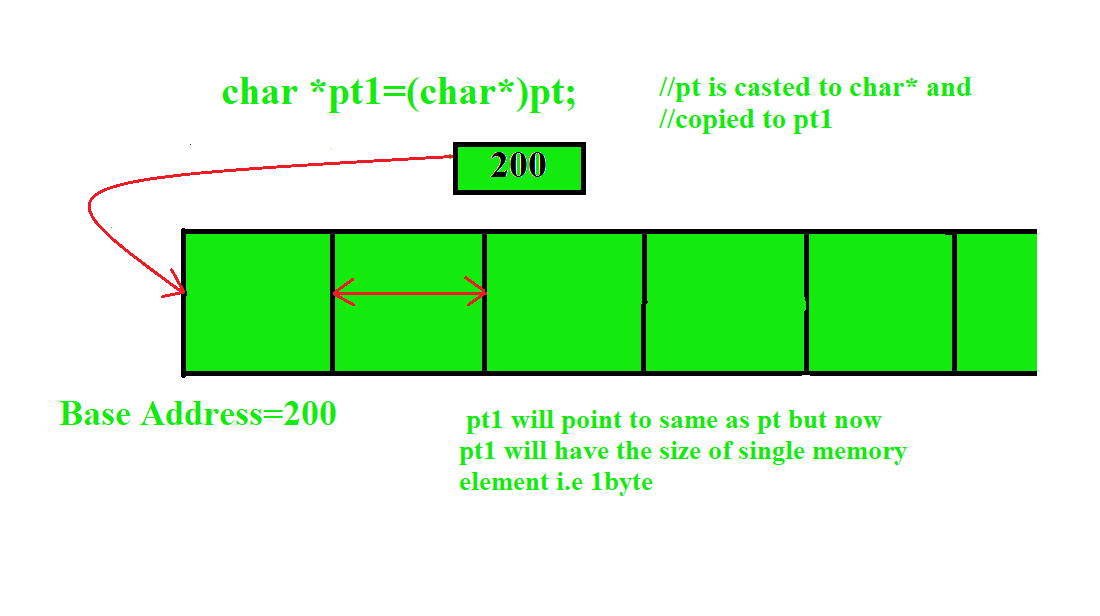GeeksforGeeks App
Open AppBrowser
Continue

# Generic Implementation of QuickSort Algorithm in C

Write a function to implement quicksort algorithm that will work for all types of data i.e ints, floats, chars etc.
It should accept all types of data and show the sorted data as output.

Note: This function is similar to C standard library function qsort().

Examples:

```First Input as a string.
Input :abc cad bcd xyz bsd
Output :abc bcd bsd cad xyz

Second input as integer
Input :5 6 4 2 3
Output :2 3 4 5 6
```

## Recommended: Please try your approach on {IDE} first, before moving on to the solution.

We use void* to implement generic quicksort function in C. void* does not know how much bytes of memory it has to occupy in memory space. It must be casted to any other data type like int*, char* before doing any operation on it.
Example: when we declare int var; compiler knows that it has occupy 4 bytes of memory but void does not know how much bytes of memory it has to occupy.
We will also use a pointer to function that will point to a function which is dependent to different types of data i.e and this function will be defined by the user according to there need.

Below is the image representation of void* in memory before and after casting it to any particular data type for better understanding.

Void* pt in Memory :void* pt casted to char* :`// C Program to illustrate Generic Quicksort Function``#include ``#include ``#include `` ` `// function for comparing two strings. This function``// is passed as a parameter to _quickSort() when we``// want to sort ``int` `cmpstr(``void``* v1, ``void``* v2)``{``    ``// casting v1 to char** and then assigning it to``    ``// pointer to v1 as v1 is array of characters i.e``    ``// strings.``    ``char` `*a1 = *(``char``**)v1;``    ``char` `*a2 = *(``char``**)v2;``    ``return` `strcmp``(a1, a2);``}`` ` `// function for comparing two strings``int` `cmpnum(``void``* s1, ``void``* s2)``{``    ``// casting s1 to int* so it can be``    ``// copied in variable a.``    ``int` `*a = (``int``*)s1;``    ``int` `*b = (``int``*)s2;``    ``if` `((*a) > (*b))``        ``return` `1;``    ``else` `if` `((*a) < (*b))``        ``return` `-1;``    ``else``        ``return` `0;``}`` ` `/* you can also write compare function for floats,``    ``chars, double similarly as integer. */``// function for swap two elements``void` `swap(``void``* v1, ``void``* v2, ``int` `size)``{``    ``// buffer is array of characters which will ``    ``// store element byte by byte``    ``char` `buffer[size];`` ` `    ``// memcpy will copy the contents from starting``    ``// address of v1 to length of size in buffer ``    ``// byte by byte.``    ``memcpy``(buffer, v1, size);``    ``memcpy``(v1, v2, size);``    ``memcpy``(v2, buffer, size);``}`` ` `// v is an array of elements to sort.``// size is the number of elements in array``// left and right is start and end of array``//(*comp)(void*, void*) is a pointer to a function``// which accepts two void* as its parameter``void` `_qsort(``void``* v, ``int` `size, ``int` `left, ``int` `right,``                      ``int` `(*comp)(``void``*, ``void``*))``{``    ``void` `*vt, *v3;``    ``int` `i, last, mid = (left + right) / 2;``    ``if` `(left >= right)``        ``return``;`` ` `    ``// casting void* to char* so that operations ``    ``// can be done.``    ``void``* vl = (``char``*)(v + (left * size));``    ``void``* vr = (``char``*)(v + (mid * size));``    ``swap(vl, vr, size);``    ``last = left;``    ``for` `(i = left + 1; i <= right; i++) {`` ` `        ``// vl and vt will have the starting address ``        ``// of the elements which will be passed to ``        ``// comp function.``        ``vt = (``char``*)(v + (i * size));``        ``if` `((*comp)(vl, vt) > 0) {``            ``++last;``            ``v3 = (``char``*)(v + (last * size));``            ``swap(vt, v3, size);``        ``}``    ``}``    ``v3 = (``char``*)(v + (last * size));``    ``swap(vl, v3, size);``    ``_qsort(v, size, left, last - 1, comp);``    ``_qsort(v, size, last + 1, right, comp);``}`` ` `int` `main()``{``    ``// Your C Code``    ``char``* a[] = {``"bbc"``, ``"xcd"``, ``"ede"``, ``"def"``,``            ``"afg"``, ``"hello"``, ``"hmmm"``, ``"okay"``, ``"how"` `};`` ` `    ``int` `b[] = { 45, 78, 89, 65, 70, 23, 44 };``    ``int``* p = b;``    ``_qsort(a, ``sizeof``(``char``*), 0, 8, (``int` `(*)(``void``*, ``void``*))(cmpstr));``    ``_qsort(p, ``sizeof``(``int``), 0, 6, (``int` `(*)(``void``*, ``void``*))(cmpnum));`` ` `    ``for` `(``int` `i = 0; i < 9; i++)``        ``printf``(``"%s "``, a[i]);``    ``printf``(``"\n"``);`` ` `    ``for` `(``int` `i = 0; i < 7; i++)``        ``printf``(``"%d "``, b[i]);``    ``return` `0;``}`

Output:

```afg bbc def ede hello hmmm how okay xcd
23 44 45 65 70 78 89
```

My Personal Notes arrow_drop_up